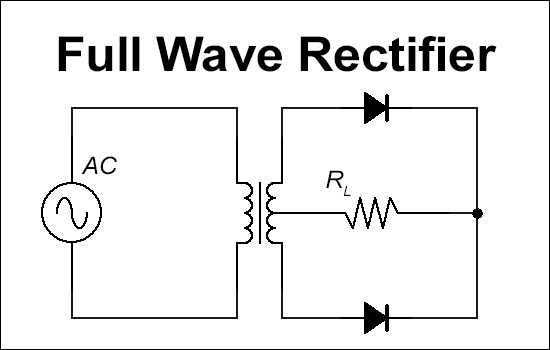# Full Wave Rectifier - Average Output Voltage and Rectifying Efficiency Calculator

This tool calculates the average output voltage and rectifying efficiency of a full wave rectifier while taking into account the forward diode resistance.

The average output voltage of a full wave rectifier when the diode resistance is zero is approximately 0.637*AC Input Voltage(max)) or 0.9*AC Input Voltage(RMS). This value decreases as the diode resistance increases. It is also affected by the load resistance when the diode resistance is not zero. Since the full wave rectifier rectifies double the amount of a half wave rectifier, the average ouput voltage is double that of a half wave rectifier in the same conditions.

The rectifying efficiency of a full wave rectifier when the forward diode resistance is zero is approximately 81.06%. Thus, the efficiency cannot exceed that value; the rectifying efficiency decreases as the diode resistance increases.

The output voltage and efficiency is rounded to the hudredths place. Please notify the admin if there are any bugs or requests.

The term "diode resistance" is equal to "forward diode resistance" in this passage.### Input

Load Resistance (RL):

Forward Diode Resistance (r):

Input AC Voltage:
Volts
RMS Voltage
Max Voltage